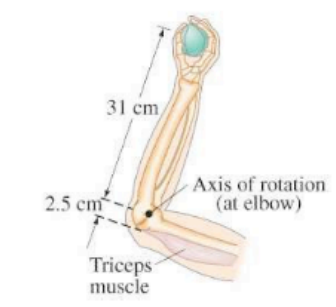×
Get Full Access to Physics: Principles With Applications - 6 Edition - Chapter 8 - Problem 38p
Get Full Access to Physics: Principles With Applications - 6 Edition - Chapter 8 - Problem 38p

×

# (II) The forearm in Fig. 8-46 accelerates a 3.6-kg ball atISBN: 9780130606204 3

## Solution for problem 38P Chapter 8

Physics: Principles with Applications | 6th Edition

• Textbook Solutions
• 2901 Step-by-step solutions solved by professors and subject experts
• Get 24/7 help from StudySoup virtual teaching assistantsPhysics: Principles with Applications | 6th Edition

4 5 1 333 Reviews
25
5
Problem 38P

(II) The forearm in Fig.accelerates aball atby means of the triceps muscle, as shown. Calcu. late (a) the torque needed, and () the force that must be exerted by the triceps muscle. Ignore the mass of the arm.FIGURE 8-45 Problem 38

Step-by-Step Solution:

Step-by-step solution

Step 1 of 3

Mass of ball m = 3.6 kg

Acceleration of ballDistance of the ball from the jointDistance between triceps muscles

Step 2 of 3

Step 3 of 3

##### ISBN: 9780130606204

The answer to “?(II) The forearm in Fig. accelerates a ball at by means of the triceps muscle, as shown. Calcu. late (a) the torque needed, and ( ) the force that must be exerted by the triceps muscle. Ignore the mass of the arm. FIGURE 8-45 38” is broken down into a number of easy to follow steps, and 45 words. Physics: Principles with Applications was written by and is associated to the ISBN: 9780130606204. Since the solution to 38P from 8 chapter was answered, more than 1809 students have viewed the full step-by-step answer. The full step-by-step solution to problem: 38P from chapter: 8 was answered by , our top Physics solution expert on 03/03/17, 03:53PM. This textbook survival guide was created for the textbook: Physics: Principles with Applications, edition: 6. This full solution covers the following key subjects: triceps, muscle, ignore, calculate, exerted. This expansive textbook survival guide covers 35 chapters, and 3914 solutions.

Unlock Textbook Solution